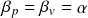# Fine-structure constant

The fine-structure constant is a dimensionless operator quantifying the reduction in charge potential from the maximum Planck charge. The operator is denoted by the Greek letter alpha

The fine-structure constant is a mysterious quantity with no theoretical explanation of its magnitude. It appears as an operator in a number of historical constants including the elementary charge, Rydberg constant, von Klitzing constant, Josephson constant, magnetic flux constant, Bohr magneton, and conductance quantum.

Electric charge is naturally quantified in units of time, where the maximum charge potential is equal to the Planck time. The elementary charge in natural units is therefore

## Atomic orbitals

The significance of the the fine-structure constant is shown in the quantization of atomic orbitals. In the language of the New Foundations Model, the fine structure constant determines the electron’s wavelength and velocity according to the relationThis relation is expressed in the elementary form of the Rydberg energy formula

which is equivalent to the New Foundations Model energy formula

In the hydrogen model of the atom, the electron’s wavelength and velocity change from orbital to orbital in increments of the fine-structure constantFine-structure constant